Similarity-Based Remaining Useful Life Estimation

This example shows how to build a complete Remaining Useful Life (RUL) estimation workflow including the steps for preprocessing, selecting trendable features, constructing a health indicator by sensor fusion, training similarity RUL estimators, and validating prognostics performance. The example uses the training data from the PHM2008 challenge dataset from https://ti.arc.nasa.gov/tech/dash/groups/pcoe/prognostic-data-repository/ .

Data Preparation

Since the dataset is small it is feasible to load the whole degradation data into memory. Download and unzip the data set from https://ti.arc.nasa.gov/tech/dash/groups/pcoe/prognostic-data-repository/ to the current directory. Use the helperLoadData helper function to load and convert the training text file to a cell array of timetables. The training data contains 218 run-to-failure simulations. This group of measurements is called an "ensemble".

ans = 5×1 cell array
{223×26 table}
{164×26 table}
{150×26 table}
{159×26 table}
{357×26 table}

Each ensemble member is a table with 26 columns. The columns contain data for the machine ID, time stamp, 3 operating conditions and 21 sensor measurements.

ans=8×26 table
id    time    op_setting_1    op_setting_2    op_setting_3    sensor_1    sensor_2    sensor_3    sensor_4    sensor_5    sensor_6    sensor_7    sensor_8    sensor_9    sensor_10    sensor_11    sensor_12    sensor_13    sensor_14    sensor_15    sensor_16    sensor_17    sensor_18    sensor_19    sensor_20    sensor_21
__    ____    ____________    ____________    ____________    ________    ________    ________    ________    ________    ________    ________    ________    ________    _________    _________    _________    _________    _________    _________    _________    _________    _________    _________    _________    _________

1      1         10.005          0.2501            20          489.05      604.13      1499.5        1310      10.52       15.49       394.88      2318.9      8770.2       1.26          45.4       372.15       2388.1       8120.8       8.6216        0.03          368         2319          100         28.58       17.174
1      2         0.0015          0.0003           100          518.67      642.13      1584.5        1404      14.62       21.61       553.67        2388      9045.8        1.3         47.29       521.81       2388.2       8132.9       8.3907        0.03          391         2388          100         38.99       23.362
1      3         34.999          0.8401            60          449.44      555.42      1368.2      1122.5       5.48           8       194.93      2222.9      8343.9       1.02         41.92       183.26       2387.9       8063.8       9.3557        0.02          334         2223          100         14.83       8.8555
1      4         20.003          0.7005             0          491.19      607.03      1488.4      1249.2       9.35       13.65       334.82      2323.8      8721.5       1.08         44.26       314.84       2388.1       8052.3       9.2231        0.02          364         2324          100         24.42       14.783
1      5         42.004          0.8405            40             445      549.52      1354.5      1124.3       3.91        5.71       138.24      2211.8      8314.6       1.02         41.79       130.44       2387.9       8083.7       9.2986        0.02          330         2212          100         10.99       6.4025
1      6         20.003          0.7017             0          491.19      607.37      1480.5      1258.9       9.35       13.65       334.51      2323.9      8711.4       1.08          44.4       315.36       2388.1       8053.2       9.2276        0.02          364         2324          100         24.44       14.702
1      7             42            0.84            40             445      549.57      1354.4      1131.4       3.91        5.71       139.11      2211.8      8316.9       1.02         42.09       130.16       2387.9         8082       9.3753        0.02          331         2212          100         10.53       6.4254
1      8         0.0011               0           100          518.67      642.08      1589.5      1407.6      14.62       21.61       553.48      2388.1      9050.4        1.3          47.5       521.74         2388       8133.3       8.4339        0.03          391         2388          100         38.98       23.234

Split the degradation data into a training data set and a validation data set for later performance evaluation.

rng('default')  % To make sure the results are repeatable
numFold = 5;
cv = cvpartition(numEnsemble, 'KFold', numFold);

Specify groups of variables of interest.

timeVariable = varNames{2};
conditionVariables = varNames(3:5);
dataVariables = varNames(6:26);

Visualize a sample of the ensemble data.

nsample = 10;
figure
helperPlotEnsemble(trainData, timeVariable, ...
[conditionVariables(1:2) dataVariables(1:2)], nsample)Working Regime Clustering

As shown in the previous section, there is no clear trend showing the degradation process in each run-to-failure measurement. In this and the next section, the operating conditions will be used to extract clearer degradation trends from sensor signals.

Notice that each ensemble member contains 3 operating conditions: "op_setting_1", "op_setting_2", and "op_setting_3". First, let's extract the table from each cell and concatenate them into a single table.

trainDataUnwrap = vertcat(trainData{:});
opConditionUnwrap = trainDataUnwrap(:, cellstr(conditionVariables));

Visualize all operating points on a 3D scatter plot. It clearly shows 6 regimes and the points in each regime are in very close proximity.

figure
helperPlotClusters(opConditionUnwrap)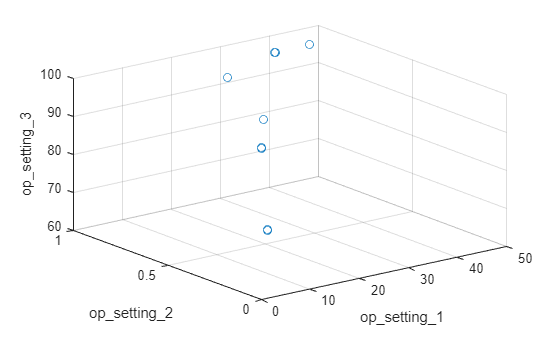Let's use clustering techniques to locate the 6 clusters automatically. Here, the K-means algorithm is used. K-means is one of the most popular clustering algorithms, but it can result in local optima. It is a good practice to repeat the K-means clustering algorithm several times with different initial conditions and pick the results with the lowest cost. In this case, the algorithm runs 5 times and the results are identical.

opts = statset('Display', 'final');
[clusterIndex, centers] = kmeans(table2array(opConditionUnwrap), 6, ...
'Distance', 'sqeuclidean', 'Replicates', 5, 'Options', opts);
Replicate 1, 1 iterations, total sum of distances = 0.279547.
Replicate 2, 1 iterations, total sum of distances = 0.279547.
Replicate 3, 1 iterations, total sum of distances = 0.279547.
Replicate 4, 1 iterations, total sum of distances = 0.279547.
Replicate 5, 1 iterations, total sum of distances = 0.279547.
Best total sum of distances = 0.279547

Visualize the clustering results and the identified cluster centroids.

figure
helperPlotClusters(opConditionUnwrap, clusterIndex, centers)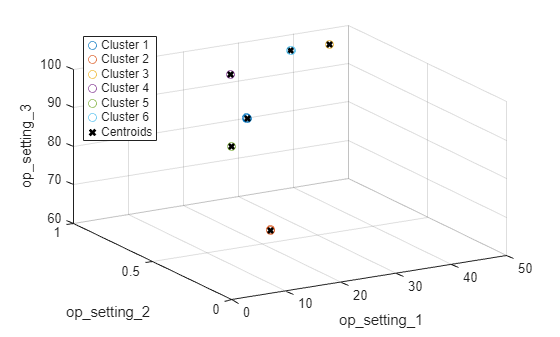As the plot illustrates, the clustering algorithm successfully finds the 6 working regimes.

Working Regime Normalization

Let's perform a normalization on measurements grouped by different working regimes. First, compute the mean and standard deviation of each sensor measurement grouped by the working regimes identified in the last section.

centerstats = struct('Mean', table(), 'SD', table());
for v = dataVariables
centerstats.Mean.(char(v)) = splitapply(@mean, trainDataUnwrap.(char(v)), clusterIndex);
centerstats.SD.(char(v))   = splitapply(@std,  trainDataUnwrap.(char(v)), clusterIndex);
end
centerstats.Mean
ans=6×21 table
sensor_1    sensor_2    sensor_3    sensor_4    sensor_5    sensor_6    sensor_7    sensor_8    sensor_9    sensor_10    sensor_11    sensor_12    sensor_13    sensor_14    sensor_15    sensor_16    sensor_17    sensor_18    sensor_19    sensor_20    sensor_21
________    ________    ________    ________    ________    ________    ________    ________    ________    _________    _________    _________    _________    _________    _________    _________    _________    _________    _________    _________    _________

489.05      604.92      1502.1      1311.4      10.52       15.493      394.32        2319      8784.1         1.26      45.496       371.44       2388.2       8133.9       8.6653          0.03      369.74        2319           100       28.525       17.115
518.67      642.71      1590.7      1409.4      14.62        21.61       553.3      2388.1      9062.3          1.3      47.557       521.36       2388.1       8140.9       8.4442          0.03      393.27        2388           100       38.809       23.285
462.54      536.87      1262.8      1050.6       7.05       9.0275       175.4      1915.4      8014.9      0.93989      36.808       164.56       2028.3         7878       10.916          0.02      307.39        1915         84.93       14.262       8.5552
445      549.72      1354.7      1128.2       3.91       5.7158      138.62        2212        8327       1.0202      42.163       130.53         2388       8088.4       9.3775          0.02      331.12        2212           100       10.584       6.3515
491.19      607.59        1486      1253.6       9.35       13.657      334.46        2324      8729.1       1.0777      44.466       314.85       2388.2         8065       9.2347      0.022299      365.45        2324           100       24.447        14.67
449.44      555.82      1366.9      1131.9       5.48       8.0003      194.43        2223      8355.2       1.0203      41.995       183.01       2388.1       8071.1       9.3344          0.02      334.29        2223           100       14.827       8.8966

centerstats.SD
ans=6×21 table
sensor_1     sensor_2    sensor_3    sensor_4     sensor_5     sensor_6     sensor_7    sensor_8    sensor_9    sensor_10     sensor_11    sensor_12    sensor_13    sensor_14    sensor_15    sensor_16     sensor_17    sensor_18    sensor_19     sensor_20    sensor_21
__________    ________    ________    ________    __________    _________    ________    ________    ________    __________    _________    _________    _________    _________    _________    __________    _________    _________    __________    _________    _________

1.4553e-11    0.47617      5.8555      8.3464     1.1618e-12    0.0047272    0.65536     0.094487     18.057       1.51e-13     0.25089      0.52838     0.096183      16.012      0.037598     9.9929e-16     1.4723          0                 0     0.14522     0.086753
4.059e-11    0.48566      5.9258      8.8223     3.7307e-14    0.0011406    0.86236     0.068654     20.061     1.2103e-13     0.25937      0.71239     0.069276      17.212      0.036504     9.9929e-16     1.5046          0                 0     0.17565      0.10515
2.7685e-11    0.35468      5.2678      6.9664      2.043e-14    0.0043301    0.45074       0.2743     14.741      0.0010469     0.21539      0.33985      0.28932      13.624      0.044142     8.6744e-16     1.3083          0        5.9833e-12     0.11212      0.06722
0    0.44169      5.6853      7.5741     1.9763e-13    0.0049401    0.44198      0.30713     18.389      0.0014951     0.23584       0.3432      0.33144      16.917      0.037135      2.231e-15     1.4174          0                 0     0.10778     0.063991
4.4456e-11    0.46992      5.7664      7.8679     8.9892e-13    0.0046633    0.59984      0.13032     17.983      0.0042388      0.2362      0.49055      0.13285      15.792      0.038156      0.0042084     1.4428          0                 0     0.13352     0.079731
4.3489e-11    0.44341      5.7224      7.4842     3.7485e-13    0.0017642     0.4734      0.28889     17.608      0.0017978     0.23242      0.38243         0.31       15.91      0.038624     8.5009e-16     1.3974          0                 0     0.11311     0.069348

The statistics in each regime can be used to normalize the training data. For each ensemble member, extract the operating points of each row, compute its distance to each cluster centers and find the nearest cluster center. Then, for each sensor measurement, subtract the mean and divide it by the standard deviation of that cluster. If the standard deviation is close to 0, set the normalized sensor measurement to 0 because a nearly constant sensor measurement is not useful for remaining useful life estimation. Refer to the last section, "Helper Functions", for more details on regimeNormalization function.

trainDataNormalized = cellfun(@(data) regimeNormalization(data, centers, centerstats), ...
trainData, 'UniformOutput', false);

Visualize the data normalized by working regime. Degradation trends for some sensor measurements are now revealed after normalization.

figure
helperPlotEnsemble(trainDataNormalized, timeVariable, dataVariables(1:4), nsample)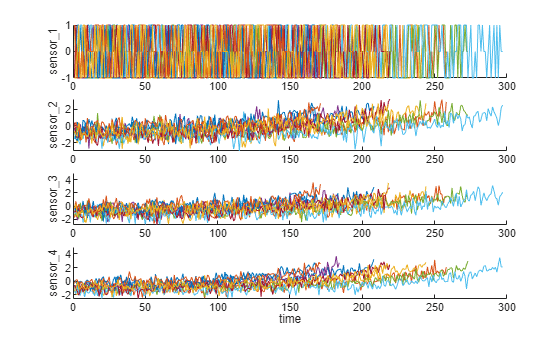Trendability Analysis

Now select the most trendable sensor measurements to construct a health indicator for prediction. For each sensor measurement, a linear degradation model is estimated and the slopes of the signals are ranked.

numSensors = length(dataVariables);
signalSlope = zeros(numSensors, 1);
warn = warning('off');
for ct = 1:numSensors
tmp = cellfun(@(tbl) tbl(:, cellstr(dataVariables(ct))), trainDataNormalized, 'UniformOutput', false);
mdl = linearDegradationModel(); % create model
fit(mdl, tmp); % train mode
signalSlope(ct) = mdl.Theta;
end
warning(warn);

Sort the signal slopes and select 8 sensors with the largest slopes.

[~, idx] = sort(abs(signalSlope), 'descend');
sensorTrended = sort(idx(1:8))
sensorTrended = 8×1

2
3
4
7
11
12
15
17

Visualize the selected trendable sensor measurements.

figure
helperPlotEnsemble(trainDataNormalized, timeVariable, dataVariables(sensorTrended(3:6)), nsample)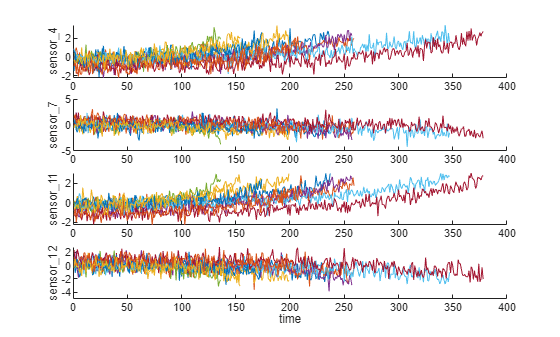Notice that some of the most trendable signals show positive trends, while others show negative trends.

Construct Health Indicator

This section focuses on fusing the sensor measurements into a single health indicator, with which a similarity-based model is trained.

All the run-to-failure data is assumed to start with a healthy condition. The health condition at the beginning is assigned a value of 1 and the health condition at failure is assigned a value of 0. The health condition is assumed to be linearly degrading from 1 to 0 over time. This linear degradation is used to help fuse the sensor values. More sophisticated sensor fusion techniques are described in the literature [2-5].

for j=1:numel(trainDataNormalized)
data = trainDataNormalized{j};
rul = max(data.time)-data.time;
data.health_condition = rul / max(rul);
trainDataNormalized{j} = data;
end

Visualize the health condition.

figure
helperPlotEnsemble(trainDataNormalized, timeVariable, "health_condition", nsample)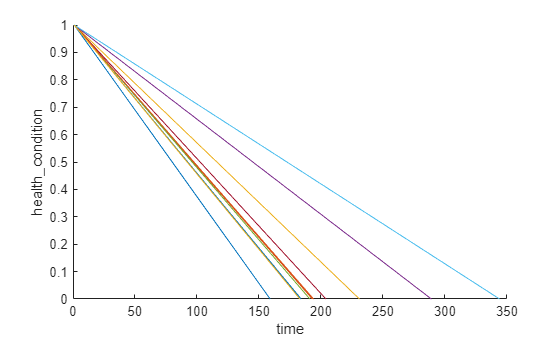The health condition of all ensemble members change from 1 to 0 with varying degrading speeds.

Now fit a linear regression model of Health Condition with the most trended sensor measurements as regressors:

Health Condition ~ 1 + Sensor2 + Sensor3 + Sensor4 + Sensor7 + Sensor11 + Sensor12 + Sensor15 + Sensor17

trainDataNormalizedUnwrap = vertcat(trainDataNormalized{:});

sensorToFuse = dataVariables(sensorTrended);
X = trainDataNormalizedUnwrap{:, cellstr(sensorToFuse)};
y = trainDataNormalizedUnwrap.health_condition;
regModel = fitlm(X,y);
bias = regModel.Coefficients.Estimate(1)
bias = 0.5000
weights = regModel.Coefficients.Estimate(2:end)
weights = 8×1

-0.0308
-0.0308
-0.0536
0.0033
-0.0639
0.0051
-0.0408
-0.0382

Construct a single health indicator by multiplying the sensor measurements with their associated weights .

trainDataFused = cellfun(@(data) degradationSensorFusion(data, sensorToFuse, weights), trainDataNormalized, ...
'UniformOutput', false);

Visualize the fused health indicator for training data.

figure
helperPlotEnsemble(trainDataFused, [], 1, nsample)
xlabel('Time')
ylabel('Health Indicator')
title('Training Data')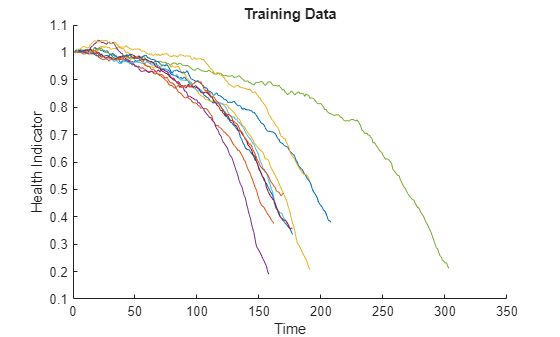The data from multiple sensors are fused into a single health indicator. The health indicator is smoothed by a moving average filter. See helper function "degradationSensorFusion" in the last section for more details.

Apply same operation to validation data

Repeat the regime normalization and sensor fusion process with the validation data set.

validationDataNormalized = cellfun(@(data) regimeNormalization(data, centers, centerstats), ...
validationData, 'UniformOutput', false);
validationDataFused = cellfun(@(data) degradationSensorFusion(data, sensorToFuse, weights), ...
validationDataNormalized, 'UniformOutput', false);

Visualize the health indicator for validation data.

figure
helperPlotEnsemble(validationDataFused, [], 1, nsample)
xlabel('Time')
ylabel('Health Indicator')
title('Validation Data')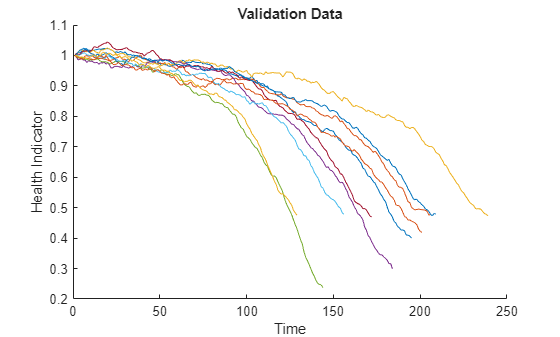Build Similarity RUL Model

Now build a residual-based similarity RUL model using the training data. In this setting, the model tries to fit each fused data with a 2nd order polynomial.

The distance between data $\mathit{i}$ and data $\mathit{j}$ is computed by the 1-norm of the residual

$d\left(i,j\right)=||{y}_{j}-{\underset{}{\overset{ˆ}{y}}}_{j,i}|{|}_{1}$

where ${\mathit{y}}_{\mathit{j}}$ is the health indicator of machine $\mathit{j}$, ${\underset{}{\overset{ˆ}{y}}}_{j,i}$ is the estimated health indicator of machine $\mathit{j}$ using the 2nd order polynomial model identified in machine $\mathit{i}$.

The similarity score is computed by the following formula

$score\left(i,j\right)=exp\left(-d\left(i,j{\right)}^{2}\right)$

Given one ensemble member in the validation data set, the model will find the nearest 50 ensemble members in the training data set, fit a probability distribution based on the 50 ensemble members, and use the median of the distribution as an estimate of RUL.

mdl = residualSimilarityModel(...
'Method', 'poly2',...
'Distance', 'absolute',...
'NumNearestNeighbors', 50,...
'Standardize', 1);

fit(mdl, trainDataFused);

Performance Evaluation

To evaluate the similarity RUL model, use 50%, 70% and 90% of a sample validation data to predict its RUL.

breakpoint = [0.5, 0.7, 0.9];
validationDataTmp = validationDataFused{3}; % use one validation data for illustration

Use the validation data before the first breakpoint, which is 50% of the lifetime.

bpidx = 1;
validationDataTmp50 = validationDataTmp(1:ceil(end*breakpoint(bpidx)),:);
trueRUL = length(validationDataTmp) - length(validationDataTmp50);
[estRUL, ciRUL, pdfRUL] = predictRUL(mdl, validationDataTmp50);

Visualize the validation data truncated at 50% and its nearest neighbors.

figure
compare(mdl, validationDataTmp50);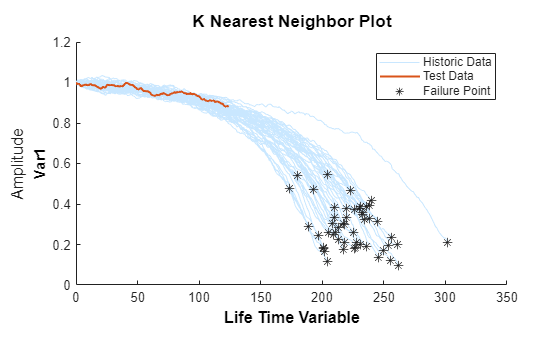Visualize the estimated RUL compared to the true RUL and the probability distribution of the estimated RUL.

figure
helperPlotRULDistribution(trueRUL, estRUL, pdfRUL, ciRUL)There is a relatively large error between the estimated RUL and the true RUL when the machine is in an intermediate health stage. In this example, the most similar 10 curves are close at the beginning, but bifurcate when they approach the failure state, resulting in roughly two modes in the RUL distribution.

Use the validation data before the second breakpoint, which is 70% of the lifetime.

bpidx = 2;
validationDataTmp70 = validationDataTmp(1:ceil(end*breakpoint(bpidx)), :);
trueRUL = length(validationDataTmp) - length(validationDataTmp70);
[estRUL,ciRUL,pdfRUL] = predictRUL(mdl, validationDataTmp70);

figure
compare(mdl, validationDataTmp70);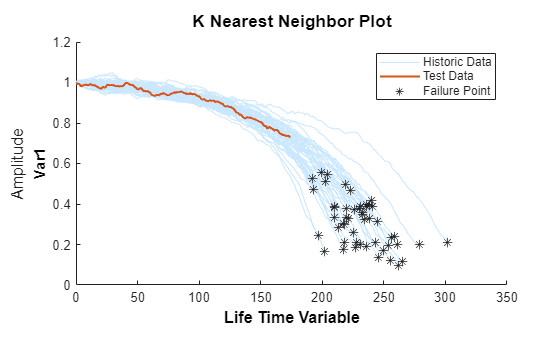figure
helperPlotRULDistribution(trueRUL, estRUL, pdfRUL, ciRUL)When more data is observed, the RUL estimation is enhanced.

Use the validation data before the third breakpoint, which is 90% of the lifetime.

bpidx = 3;
validationDataTmp90 = validationDataTmp(1:ceil(end*breakpoint(bpidx)), :);
trueRUL = length(validationDataTmp) - length(validationDataTmp90);
[estRUL,ciRUL,pdfRUL] = predictRUL(mdl, validationDataTmp90);

figure
compare(mdl, validationDataTmp90);figure
helperPlotRULDistribution(trueRUL, estRUL, pdfRUL, ciRUL)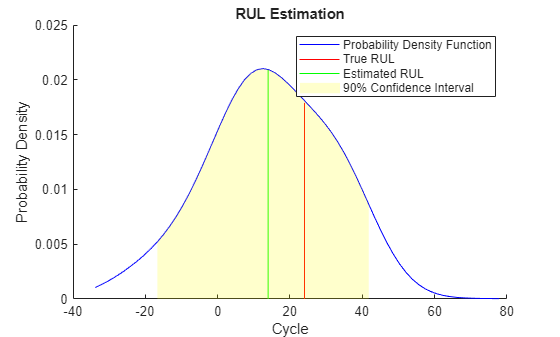When the machine is close to failure, the RUL estimation is even more enhanced in this example.

Now repeat the same evaluation procedure for the whole validation data set and compute the error between estimated RUL and true RUL for each breakpoint.

numValidation = length(validationDataFused);
numBreakpoint = length(breakpoint);
error = zeros(numValidation, numBreakpoint);

for dataIdx = 1:numValidation
tmpData = validationDataFused{dataIdx};
for bpidx = 1:numBreakpoint
tmpDataTest = tmpData(1:ceil(end*breakpoint(bpidx)), :);
trueRUL = length(tmpData) - length(tmpDataTest);
[estRUL, ~, ~] = predictRUL(mdl, tmpDataTest);
error(dataIdx, bpidx) = estRUL - trueRUL;
end
end

Visualize the histogram of the error for each breakpoint together with its probability distribution.

[pdf50, x50] = ksdensity(error(:, 1));
[pdf70, x70] = ksdensity(error(:, 2));
[pdf90, x90] = ksdensity(error(:, 3));

figure
ax(1) = subplot(3,1,1);
hold on
histogram(error(:, 1), 'BinWidth', 5, 'Normalization', 'pdf')
plot(x50, pdf50)
hold off
xlabel('Prediction Error')
title('RUL Prediction Error using first 50% of each validation ensemble member')

ax(2) = subplot(3,1,2);
hold on
histogram(error(:, 2), 'BinWidth', 5, 'Normalization', 'pdf')
plot(x70, pdf70)
hold off
xlabel('Prediction Error')
title('RUL Prediction Error using first 70% of each validation ensemble member')

ax(3) = subplot(3,1,3);
hold on
histogram(error(:, 3), 'BinWidth', 5, 'Normalization', 'pdf')
plot(x90, pdf90)
hold off
xlabel('Prediction Error')
title('RUL Prediction Error using first 90% of each validation ensemble member')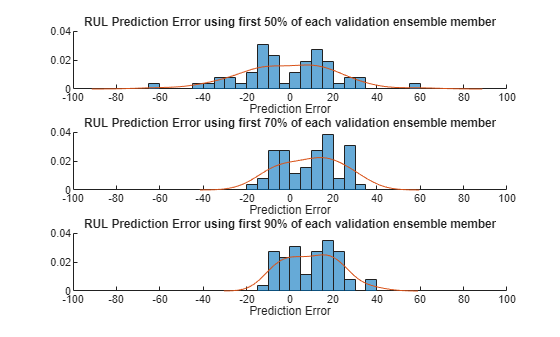Plot the prediction error in a box plot to visualize the median, 25-75 quantile and outliers.

figure
boxplot(error, 'Labels', {'50%', '70%', '90%'})
ylabel('Prediction Error')
title('Prediction error using different percentages of each validation ensemble member')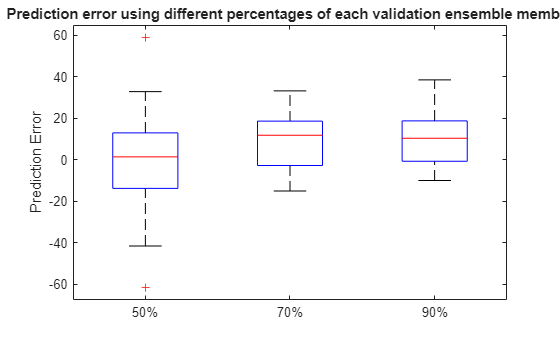Compute and visualize the mean and standard deviation of the prediction error.

errorMean = mean(error)
errorMean = 1×3

-5.8944    3.1359    3.3555

errorMedian = median(error)
errorMedian = 1×3

-4.8538    5.3763    3.6580

errorSD = std(error)
errorSD = 1×3

26.4916   20.0720   18.0313

figure
errorbar([50 70 90], errorMean, errorSD, '-o', 'MarkerEdgeColor','r')
xlim([40, 100])
xlabel('Percentage of validation data used for RUL prediction')
ylabel('Prediction Error')
legend('Mean Prediction Error with 1 Standard Deviation Eror bar', 'Location', 'south')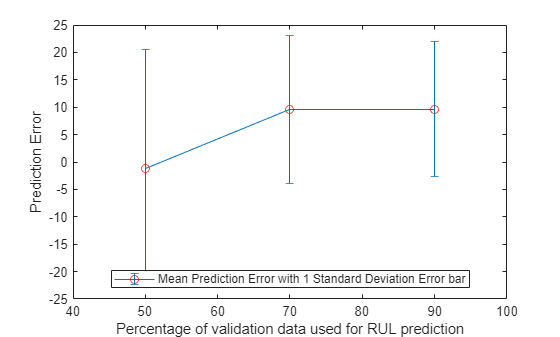It is shown that the error becomes more concentrated around 0 (less outliers) as more data is observed.

References

 A. Saxena and K. Goebel (2008). "PHM08 Challenge Data Set", NASA Ames Prognostics Data Repository (http://ti.arc.nasa.gov/project/prognostic-data-repository), NASA Ames Research Center, Moffett Field, CA

 Roemer, Michael J., Gregory J. Kacprzynski, and Michael H. Schoeller. "Improved diagnostic and prognostic assessments using health management information fusion." AUTOTESTCON Proceedings, 2001. IEEE Systems Readiness Technology Conference. IEEE, 2001.

 Goebel, Kai, and Piero Bonissone. "Prognostic information fusion for constant load systems." Information Fusion, 2005 8th International Conference on. Vol. 2. IEEE, 2005.

 Wang, Peng, and David W. Coit. "Reliability prediction based on degradation modeling for systems with multiple degradation measures." Reliability and Maintainability, 2004 Annual Symposium-RAMS. IEEE, 2004.

 Jardine, Andrew KS, Daming Lin, and Dragan Banjevic. "A review on machinery diagnostics and prognostics implementing condition-based maintenance." Mechanical systems and signal processing 20.7 (2006): 1483-1510.

Helper Functions

function data = regimeNormalization(data, centers, centerstats)
% Perform normalization for each observation (row) of the data
% according to the cluster the observation belongs to.
conditionIdx = 3:5;
dataIdx = 6:26;

% Perform row-wise operation
data{:, dataIdx} = table2array(...
rowfun(@(row) localNormalize(row, conditionIdx, dataIdx, centers, centerstats), ...
data, 'SeparateInputs', false));
end

function rowNormalized = localNormalize(row, conditionIdx, dataIdx, centers, centerstats)
% Normalization operation for each row.

% Get the operating points and sensor measurements
ops = row(1, conditionIdx);
sensor = row(1, dataIdx);

% Find which cluster center is the closest
dist = sum((centers - ops).^2, 2);
[~, idx] = min(dist);

% Normalize the sensor measurements by the mean and standard deviation of the cluster.
% Reassign NaN and Inf to 0.
rowNormalized = (sensor - centerstats.Mean{idx, :}) ./ centerstats.SD{idx, :};
rowNormalized(isnan(rowNormalized) | isinf(rowNormalized)) = 0;
end

function dataFused = degradationSensorFusion(data, sensorToFuse, weights)
% Combine measurements from different sensors according
% to the weights, smooth the fused data and offset the data
% so that all the data start from 1

% Fuse the data according to weights
dataToFuse = data{:, cellstr(sensorToFuse)};
dataFusedRaw = dataToFuse*weights;

% Smooth the fused data with moving mean
stepBackward = 10;
stepForward = 10;
dataFused = movmean(dataFusedRaw, [stepBackward stepForward]);

% Offset the data to 1
dataFused = dataFused + 1 - dataFused(1);
end